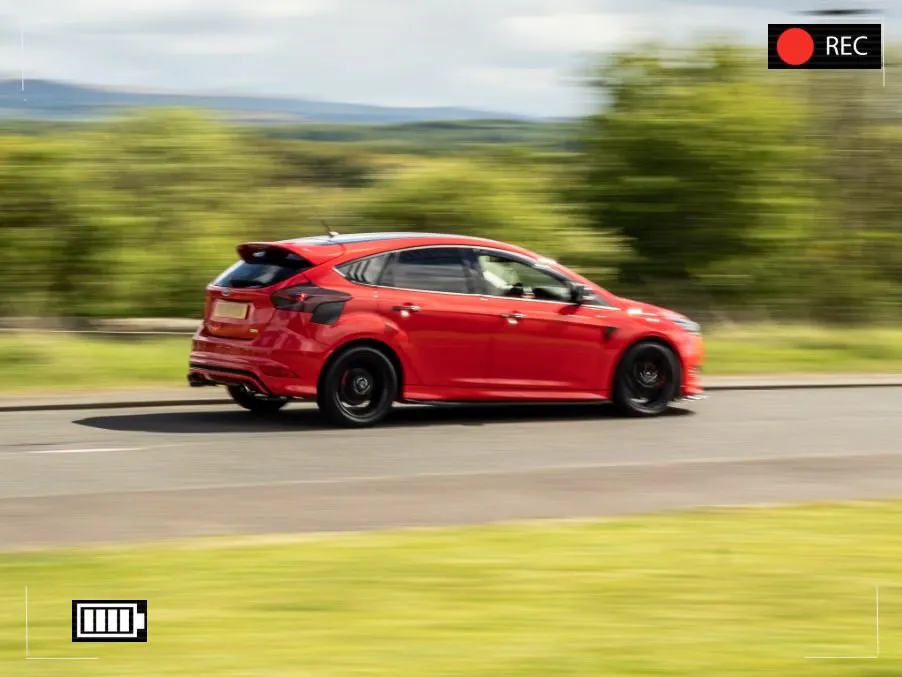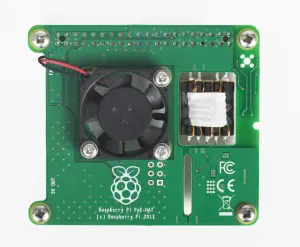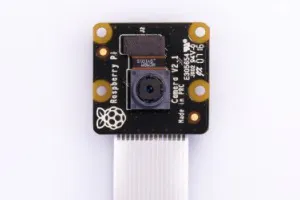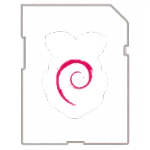# Traffic camera

An outdoor camera that counts passing traffic and logs their speed and direction. See the results at www.burnshotroad.org

IntermediateFull instructions provided3 days9,214## Things used in this project

### Hardware componentsRaspberry Pi 3 Model B
×1Raspberry Pi PoE HAT
×1Raspberry Pi Pi NoIR Camera V2
×1
 Naturebytes Wildlife Cam Case
×1
 5v Cooling Fan for Raspberry Pi Doesn't have to be this model - any fan compatible with the Pi will do. This one also included a heat sink to place on the CPU.
×1

### Software apps and online servicesOpenCVRaspberry Pi Raspbian

## Code

### carspeed Version 3

Python
Example python3 carspeed.py -ulx 136 -uly 321 -lrx 896 -lry 444
```# CarSpeed Version 3.0

# import the necessary packages
from picamera.array import PiRGBArray
from picamera import PiCamera
import time
import math
import datetime
import cv2
import paho.mqtt.client as mqtt
import numpy as np
import argparse

# place a prompt on the displayed image
def prompt_on_image(txt):
global image
cv2.putText(image, txt, (10, 35),
cv2.FONT_HERSHEY_SIMPLEX, 0.35, (0, 0, 255), 1)

# calculate speed from pixels and time
def get_speed(pixels, ftperpixel, secs):
if secs > 0.0:
return ((pixels * ftperpixel)/ secs) * 0.681818    # Magic number to convert fps to mph
else:
return 0.0

# calculate elapsed seconds
def secs_diff(endTime, begTime):
diff = (endTime - begTime).total_seconds()
return diff

# record speed in .csv format
def record_speed(res):
global csvfileout
f = open(csvfileout, 'a')
f.write(res+"\n")
f.close

# mapping function equivalent to C map function from Arduino
def my_map(x, in_min, in_max, out_min, out_max):
return int((x-in_min) * (out_max-out_min) / (in_max-in_min) + out_min)

def measure_light(hsvImg):
#Determine luminance level of monitored area
#returns the median from the histogram which contains 0 - 255 levels
hist = cv2.calcHist([hsvImg], , None, ,[0,255])
windowsize = (hsvImg.size)/3   #There are 3 pixels per HSV value
count = 0
sum = 0
print (windowsize)
for value in hist:
sum = sum + value
count +=1
if (sum > windowsize/2):   #test for median
break
return count

#Reciprocal function curve to give a smaller number for bright light and a bigger number for low light
def get_save_buffer(light):
save_buffer = int((100/(light - 0.5)) + MIN_SAVE_BUFFER)
print(" save buffer " + str(save_buffer))
return save_buffer

def get_min_area(light):
if (light > 10):
light = 10;
area =int((1000 * math.sqrt(light - 1)) + 100)
print("min area= " + str(area))
return area

def get_threshold(light):
#Threshold for dark needs to be high so only pick up lights on vehicle
if (light <= 1):
threshold = 130
elif(light <= 2):
threshold = 100
elif(light <= 3):
threshold = 60
else:
threshold = THRESHOLD
print("threshold= " + str(threshold))
return threshold

def store_image():
# timestamp the image -
global cap_time, image, mean_speed
cv2.putText(image, cap_time.strftime("%A %d %B %Y %I:%M:%S%p"),
(10, image.shape - 10), cv2.FONT_HERSHEY_SIMPLEX, 0.75, (0, 255, 0), 1)
# write the speed: first get the size of the text
size, base = cv2.getTextSize( "%.0f mph" % mean_speed, cv2.FONT_HERSHEY_SIMPLEX, 2, 3)
# then center it horizontally on the image
cntr_x = int((IMAGEWIDTH - size) / 2)
cv2.putText(image, "%.0f mph" % mean_speed,
(cntr_x , int(IMAGEHEIGHT * 0.2)), cv2.FONT_HERSHEY_SIMPLEX, 2.00, (0, 255, 0), 3)
# and save the image to disk
imageFilename = "car_at_" + cap_time.strftime("%Y%m%d_%H%M%S") + ".jpg"
cv2.imwrite(imageFilename,image)

def store_traffic_data():
global cap_time, mean_speed, direction, counter, sd, client

csvString=(cap_time.strftime("%Y-%m-%d") + ' ' +\
cap_time.strftime('%H:%M:%S:%f')+','+("%.0f" % mean_speed) + ',' +\
("%d" % direction) + ',' + ("%d" % counter) + ','+ ("%d" % sd))

record_speed(csvString)

jsonstring = '{"created_at":'+'"'+ cap_time.strftime("%Y-%m-%d")+\
' ' + cap_time.strftime('%H:%M:%S:%f')+'"' +\
',"field1":'+("%.0f" % mean_speed)+\
',"field2":' + ("%d" % direction) +\
',"field3":' + ("%d" % counter) +\
',"field4":' + ("%.0f" % sd) +\
'}'
client.publish('traffic', jsonstring)  #Publish MQTT data

# define some constants
L2R_DISTANCE = 47  #<---- enter your distance-to-road value for cars going left to right here
R2L_DISTANCE = 37  #<---- enter your distance-to-road value for cars going left to right here
MIN_SPEED_IMAGE = 50  #<---- enter the minimum speed for saving images
SAVE_CSV = True  #<---- record the results in .csv format in carspeed_(date).csv
MIN_SPEED_SAVE = 10  #<---- enter the minimum speed for publishing to MQTT broker and saving to CSV
MAX_SPEED_SAVE = 80  #<---- enter the maximum speed for publishing to MQTT broker and saving to CSV

THRESHOLD = 25
MIN_AREA = 175
BLURSIZE = (15,15)
IMAGEWIDTH = 1024
IMAGEHEIGHT = 592
RESOLUTION = [IMAGEWIDTH,IMAGEHEIGHT]
FOV = 62.2 # Pi Camera v2 is wider
FPS = 30
SHOW_BOUNDS = True
SHOW_IMAGE = True

# the following enumerated values are used to make the program more readable
WAITING = 0
TRACKING = 1
SAVING = 2
UNKNOWN = 0
LEFT_TO_RIGHT = 1
RIGHT_TO_LEFT = 2
TOO_CLOSE = 0.4
MIN_SAVE_BUFFER = 2

# construct the argument parser and parse the arguments
ap = argparse.ArgumentParser()
help="upper left x coord")
help="upper left y coord")
help="lower right x coord")
help="lower right y coord")
args = vars(ap.parse_args())

upper_left_x = int(args["upper_left_x"]);
upper_left_y = int(args["upper_left_y"]);
lower_right_x = int(args["lower_right_x"]);
lower_right_y = int(args["lower_right_y"]);

# calculate the the width of the image at the distance specified
l2r_ftperpixel = l2r_frame_width_ft / float(IMAGEWIDTH)
r2l_ftperpixel = r2l_frame_width_ft / float(IMAGEWIDTH)
print("L2R Image width in feet {} at {} from camera".format("%.0f" % l2r_frame_width_ft,"%.0f" % L2R_DISTANCE))
print("R2L Image width in feet {} at {} from camera".format("%.0f" % r2l_frame_width_ft,"%.0f" % R2L_DISTANCE))

# state maintains the state of the speed computation process
# if starts as WAITING
# the first motion detected sets it to TRACKING

# if it is tracking and no motion is found or the x value moves
# out of bounds, state is set to SAVING and the speed of the object
# is calculated
# initial_x holds the x value when motion was first detected
# last_x holds the last x value before tracking was was halted
# depending upon the direction of travel, the front of the
# vehicle is either at x, or at x+w
# (tracking_end_time - tracking_start_time) is the elapsed time
# from these the speed is calculated and displayed

#Initialisation
state = WAITING
direction = UNKNOWN
initial_x = 0
last_x = 0
#initialise.
cap_time = datetime.datetime.now()
#pixel width at left and right of window to detect end of tracking
savebuffer = MIN_SAVE_BUFFER

#-- other values used in program
base_image = None
abs_chg = 0
mph = 0
secs = 0.0
ix,iy = -1,-1
fx,fy = -1,-1
drawing = False
setup_complete = False
tracking = False
text_on_image = 'No cars'
prompt = ''
save_image = False
t1 = 0.0  #timer
t2 = 0.0  #timer
lightlevel = 0

# initialise the camera.
camera = PiCamera()
camera.resolution = RESOLUTION
camera.framerate = FPS
camera.vflip = False
camera.hflip = False

rawCapture = PiRGBArray(camera, size=camera.resolution)
# allow the camera to warm up
time.sleep(0.9)

# create an image window and place it in the upper left corner of the screen
cv2.namedWindow("Speed Camera")
cv2.moveWindow("Speed Camera", 10, 40)

#Create MQTT client and connect
client = mqtt.Client('traffic_cam')
client.loop_start()

if SAVE_CSV:
csvfileout = "carspeed_{}.csv".format(datetime.datetime.now().strftime("%Y%m%d_%H%M"))
record_speed('DateTime,Speed,Direction, Counter,SD, Image,')
else:
csvfileout = ''

monitored_width = lower_right_x - upper_left_x
monitored_height = lower_right_y - upper_left_y

print("Monitored area:")
print(" upper_left_x {}".format(upper_left_x))
print(" upper_left_y {}".format(upper_left_y))
print(" lower_right_x {}".format(lower_right_x))
print(" lower_right_y {}".format(lower_right_y))
print(" monitored_width {}".format(monitored_width))
print(" monitored_height {}".format(monitored_height))
print(" monitored_area {}".format(monitored_width * monitored_height))

# capture frames from the camera (using capture_continuous.
#   This keeps the picamera in capture mode - it doesn't need
#   to prep for each frame's capture.
for frame in camera.capture_continuous(rawCapture, format="bgr", use_video_port=True):
# grab the raw NumPy array representing the image
image = frame.array

# crop area defined by [y1:y2,x1:x2]
gray = image[upper_left_y:lower_right_y,upper_left_x:lower_right_x]
# capture colour for later when measuring light levels
hsv = cv2.cvtColor(gray, cv2.COLOR_BGR2HSV)
# convert the frame to grayscale, and blur it
gray = cv2.cvtColor(gray, cv2.COLOR_BGR2GRAY)
gray = cv2.GaussianBlur(gray, BLURSIZE, 0)

# if the base image has not been defined, initialize it
if base_image is None:
base_image = gray.copy().astype("float")
rawCapture.truncate(0)
cv2.imshow("Speed Camera", image)

if lightlevel == 0:   #First pass through only
#Set threshold and min area and save_buffer based on light readings
lightlevel = my_map(measure_light(hsv),0,256,1,10)
print("light level = " + str(lightlevel))
last_lightlevel = lightlevel

# compute the absolute difference between the current image and
# base image and then turn eveything lighter gray than THRESHOLD into
# white
frameDelta = cv2.absdiff(gray, cv2.convertScaleAbs(base_image))
thresh = cv2.threshold(frameDelta, adjusted_threshold, 255, cv2.THRESH_BINARY)

# dilate the thresholded image to fill in any holes, then find contours
# on thresholded image
thresh = cv2.dilate(thresh, None, iterations=2)
(_, cnts, _) = cv2.findContours(thresh.copy(), cv2.RETR_EXTERNAL,cv2.CHAIN_APPROX_SIMPLE)

# look for motion
motion_found = False
biggest_area = 0
# examine the contours, looking for the largest one
for c in cnts:
(x1, y1, w1, h1) = cv2.boundingRect(c)
# get an approximate area of the contour
found_area = w1*h1
# find the largest bounding rectangle
if (found_area > adjusted_min_area) and (found_area > biggest_area):
biggest_area = found_area
motion_found = True
x = x1
y = y1
h = h1
w = w1
#record the timestamp at the point in code where motion found
timestamp = datetime.datetime.now()

if motion_found:
if state == WAITING:
# intialize tracking
state = TRACKING
initial_x = x
last_x = x
#if initial capture straddles start line then the
# front of vehicle is at position w when clock started
initial_w = w
initial_time = timestamp
last_mph = 0

#Initialise array for storing speeds
speeds = np.array([])
sd=0  #Initialise standard deviation

text_on_image = 'Tracking'
counter = 0   # use to test later if saving with too few data points
car_gap = secs_diff(initial_time, cap_time)
print("initial time = "+str(initial_time) + " " + "cap_time =" + str(cap_time) + " gap= " +\
str(car_gap) + " initial x= " + str(initial_x) + " initial_w= " + str(initial_w))
print(text_on_image)
print("x-chg    Secs      MPH  x-pos width  BA DIR Count time")
# if gap between cars too low then probably seeing tail lights of current car
#but I might need to tweek this if find I'm not catching fast cars
if (car_gap<TOO_CLOSE):
state = WAITING
print("too close")
else:  #state != WAITING
# compute the lapsed time
secs = secs_diff(timestamp,initial_time)
if secs >= 3: # Object taking too long to move across
state = WAITING
direction = UNKNOWN
text_on_image = 'No Car Detected'
motion_found = False
biggest_area = 0
rawCapture.truncate(0)
base_image = None
print('Resetting')
continue

if state == TRACKING:
if x >= last_x:
direction = LEFT_TO_RIGHT
abs_chg = (x + w) - (initial_x + initial_w)
mph = get_speed(abs_chg,l2r_ftperpixel,secs)
else:
direction = RIGHT_TO_LEFT
abs_chg = initial_x - x
mph = get_speed(abs_chg,r2l_ftperpixel,secs)

counter+=1   #Increment counter

speeds = np.append(speeds, mph)   #Append speed to array

if mph < 0:
print("negative speed - stopping tracking"+ "{0:7.2f}".format(secs))
if direction == LEFT_TO_RIGHT:
direction = RIGHT_TO_LEFT  #Reset correct direction
x=1  #Force save
else:
direction = LEFT_TO_RIGHT  #Reset correct direction
x=monitored_width + MIN_SAVE_BUFFER  #Force save
else:
print("{0:4d}  {1:7.2f}  {2:7.0f}   {3:4d}  {4:4d} {5:4d} {6:1d} {7:1d} {8:%H%M%S%f}". \
format(abs_chg,secs,mph,x,w,biggest_area, direction,counter, timestamp))

real_y = upper_left_y + y
real_x = upper_left_x + x

# is front of object outside the monitired boundary? Then write date, time and speed on image
# and save it
if ((x <= adjusted_save_buffer) and (direction == RIGHT_TO_LEFT)) \
or ((x+w >= monitored_width - adjusted_save_buffer) \
and (direction == LEFT_TO_RIGHT)):

#you need at least 2 data points to calculate a mean and we're deleting one on line below
if (counter > 2):
mean_speed = np.mean(speeds[:-1])   #Mean of all items except the last one
sd = np.std(speeds[:-1])  #SD of all items except the last one
elif (counter > 1):
mean_speed = speeds[-1] # use the last element in the array
sd = 99 # Set it to a very high value to highlight it's not to be trusted.
else:
mean_speed = 0 #ignore it
sd = 0

print("numpy mean= " + "%.0f" % mean_speed)
print("numpy SD = " + "%.0f" % sd)

#Captime used for mqtt, csv, image filename.
cap_time = datetime.datetime.now()

# save the image but only if there is light and above the min speed for images
if (mean_speed > MIN_SPEED_IMAGE) and (lightlevel > 1) :
store_image()

# save the data if required and above min speed for data
if SAVE_CSV and mean_speed > MIN_SPEED_SAVE and mean_speed < MAX_SPEED_SAVE:
store_traffic_data()

counter = 0
state = SAVING
print("saving")  #debug
# if the object hasn't reached the end of the monitored area, just remember the speed
# and its last position
last_mph = mph
last_x = x
else:
# No motion detected
if state == TRACKING:
#Last frame has skipped the buffer zone
if (counter > 2):
mean_speed = np.mean(speeds[:-1])   #Mean of all items except the last one
sd = np.std(speeds[:-1])  #SD of all items except the last one
print("missed but saving")
elif (counter > 1):
mean_speed = speeds[-1] # use the last element in the array
sd = 99 # Set it to a very high value to highlight it's not to be trusted.
print("missed but saving")
else:
mean_speed = 0 #ignore it
sd = 0

print("numpy mean= " + "%.0f" % mean_speed)
print("numpy SD = " + "%.0f" % sd)

cap_time = datetime.datetime.now()
if (mean_speed > MIN_SPEED_IMAGE) and (lightlevel > 1) :
store_image()
if SAVE_CSV and mean_speed > MIN_SPEED_SAVE:
store_traffic_data()

if state != WAITING:
state = WAITING
direction = UNKNOWN
text_on_image = 'No Car Detected'
counter = 0
print(text_on_image)

# only update image and wait for a keypress when waiting for a car
# This is required since waitkey slows processing.
if (state == WAITING):

# draw the text and timestamp on the frame
cv2.putText(image, datetime.datetime.now().strftime("%A %d %B %Y %I:%M:%S%p"),
(10, image.shape - 10), cv2.FONT_HERSHEY_SIMPLEX, 0.75, (0, 255, 0), 1)
cv2.putText(image, "Road Status: {}".format(text_on_image), (10, 20),
cv2.FONT_HERSHEY_SIMPLEX,0.35, (0, 0, 255), 1)

if SHOW_BOUNDS:
#define the monitored area right and left boundary
cv2.line(image,(upper_left_x,upper_left_y),(upper_left_x,lower_right_y),(0, 255, 0))
cv2.line(image,(lower_right_x,upper_left_y),(lower_right_x,lower_right_y),(0, 255, 0))

# show the frame and check for a keypress
if SHOW_IMAGE:
prompt_on_image(prompt)
cv2.imshow("Speed Camera", image)

# Adjust the base_image as lighting changes through the day
if state == WAITING:
last_x = 0
cv2.accumulateWeighted(gray, base_image, 0.25)
t2 = time.process_time()
if (t2 - t1) > 60:   # We need to measure light level every so often
t1 = time.process_time()
lightlevel = my_map(measure_light(hsv),0,256,1,10)
print("light level = " + str(lightlevel))
if lightlevel != last_lightlevel:
base_image = None
last_lightlevel = lightlevel
state=WAITING
key = cv2.waitKey(1) & 0xFF

# if the `q` key is pressed, break from the loop and terminate processing
if key == ord("q"):
client.loop_stop()
client.disconnect()   ##disconnect from mqtt broker
break

# clear the stream in preparation for the next frame
rawCapture.truncate(0)
# cleanup the camera and close any open windows
cv2.destroyAllWindows()
```

### Carspeed version 3

Code to run Raspberry Pi with camera and count traffic and vehicle speeds. Launch with python3 carspeed.py -ulx 64 -uly 321 -lrx 960 -lry 444

## Credits

### Tim Hodges

1 project • 0 followers